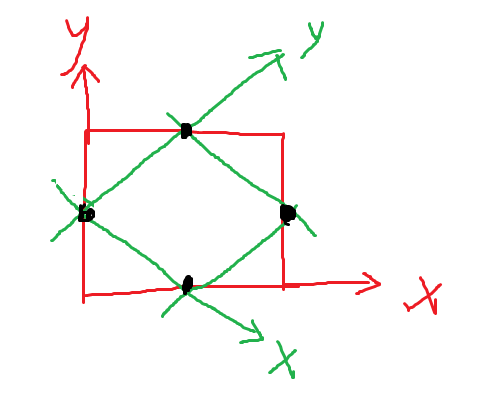# vtkTransformPolyDataFilter output a incorrect result

I have a polydata and a transformMatrix. I compute two result:

1. transform the center point of polydata with transformMatrix.
2. transform the polydata with transformMatrix, and get center of the new poly data.

I find the two results are different:

1. (32.655436515808105, 101.52645111083984, -17.70375633239746)
2. (32.64958572387695, 101.52920913696289, -17.70375633239746)


It make me confuzed.

The code to reproduce my problem is:


import vtkmodules.all as vtk

polyCenter = poly.GetCenter()

m = [
0.99999657608723425, 0.0026168327818937172, 0, -0.26556593613022272,
-0.0026168327818937172, 0.99999657608723425, 0, 0.085801434493632200,
0, 0, 1, 0,
0, 0, 0, 1
]
matrix = vtk.vtkMatrix4x4()
matrix.DeepCopy(m)
transform = vtk.vtkTransform()
transform.SetMatrix(m)
transform.Update()

print(transform.TransformPoint(polyCenter))

filter = vtk.vtkTransformPolyDataFilter()
filter.SetInputData(poly)
filter.SetTransform(transform)
filter.Update()

newPoly = filter.GetOutput()
print(newPoly.GetCenter())


And the test data is:

poly.stl (44.8 KB)

My vtk is 9.2.4

Any suggestion is appreciated~~~

The vtkSTLReader is single-precision, and this precision is carried through the transform filter. You can get more precision like this:

filter.SetOutputPointsPrecision(vtk.vtkAlgorithm.DOUBLE_PRECISION)


It don’t change the finally result. The modified code is:

filter = vtk.vtkTransformPolyDataFilter()
filter.SetInputData(poly)
filter.SetTransform(transform)
filter.SetOutputPointsPrecision(vtk.vtkAlgorithm.DOUBLE_PRECISION)
filter.Update()


And the printed information is:

(32.655436515808105, 101.52645111083984, -17.70375633239746)
(32.649586295755405, 101.52921088196327, -17.70375633239746)


The two center points are still not equal.

Ah, sorry. The cause of the difference is more fundamental than the precision of the points.

The method GetCenter() returns the center of the BoundingBox of the data. When you rotate the data, the bounding box changes.

Maybe you want the center-of-mass instead of the center of the bounding box?

How to obtain the center of mass?

I think the following points should be the same, am I right?

1. rotate the polydata with the matrix, and obtain the center of new poly data.
2. generate a actor, and set the matrix as the user matrix. Then obtain the center of actor.

However, in my test, they are still not the same:

(32.655436515808105, 101.52645111083984, -17.70375633239746)
(32.64958572387695, 101.52920913696289, -17.70375633239746)


The code to reproduce my result is:


import vtkmodules.all as vtk

polyCenter = poly.GetCenter()

m = [
0.99999657608723425, 0.0026168327818937172, 0, -0.26556593613022272,
-0.0026168327818937172, 0.99999657608723425, 0, 0.085801434493632200,
0, 0, 1, 0,
0, 0, 0, 1
]
matrix = vtk.vtkMatrix4x4()
matrix.DeepCopy(m)

mapper1 = vtk.vtkPolyDataMapper()
mapper1.SetInputData(poly)
actor1 = vtk.vtkActor()
actor1.SetMapper(mapper1)
actor1.SetUserMatrix(matrix)
actor1.Modified()

print(actor1.GetCenter())

transform = vtk.vtkTransform()
transform.SetMatrix(m)
transform.Update()

filter = vtk.vtkTransformPolyDataFilter()
filter.SetInputData(poly)
filter.SetTransform(transform)
filter.Update()

newPoly = filter.GetOutput()
print(newPoly.GetCenter())


The center of mass of the points can be computed with vtkCenterOfMass. But if you need the center of mass of the enclosed volume, I don’t think VTK provides any methods for that.

The bounds of the actor are computed by rotating the bounds of the data and then computing a new bounding box with respect to world coordinates. My feeling is that it should give the same result, but I would have to examine the code and go through the math to be certain.

However, please note that GetCenter() is only meant to be a quick-and-dirty method to get the center of the dataset. That’s why it just uses the bounding box instead of doing a more sophisticated computation.

In fact, I find the actor.SetUserMatrix() is different from newPoly=vtkTransformPolyDataFilter.GetOutput(); actor2=generateActor(newPoly). In order to debug this problem, I compute the center.

Note that “I would have to” does not have the same meaning as “I will”.

I rethink about this process. I think the bounds of the actor may be not equal to the bounds of rotated polydata.Please have a look at the above figure. Suppose we have four points (black point), and it formed a square with 1 length of side.

1. Firstly, the red line indicates the coordinate system. In this situation, the length of bounds side should be \sqrt 2.
2. Then, the matrix rotate the first coordiante system (red line) to the second coordinate system (gren line). After the rotation, the bounds of the data is rotated, and the rotated bounds should has \sqrt 2 length of side, because rotation do not change the length. To calculate the new bounding box, the bounds length should be larger than \sqrt 2.
3. However, for the rotated poly data, the bounds length should be 1.

In a word, to calculate the bounds of actor, directly rotating the bounds should be an inaccurate method.

But since the bounds are rectangular, the center should stay the same.

The main idea, though, is that GetCenter() is only meant to give an approximate result. That is why I recommend using a center-of-mass to test vtkTransformPolyDataFilter.

If you want to test the matrix of the actor, you cannot do this by computing the center of the data. This is because the transformation of the actor occurs on the GPU via OpenGL. To test the matrix of the actor, the best method is to render two actors and visually compare the results:

• for the first actor, use the actor’s matrix for the transformation
• for the second actor, use vtkTransformPolyDataFilter for the transformation

Use actor.GetProperty().SetRepresentationToWireframe() to make it easier to compare the results, and use a different color for each actor. In this way, you should be able to convince yourself that the transformation is accurate.

I have tried it. The shown actors are the same, but the obtained centers are not the same.